Courses

# Vibration Isolation Notes | EduRev

## Mechanical Engineering : Vibration Isolation Notes | EduRev

The document Vibration Isolation Notes | EduRev is a part of the Mechanical Engineering Course Theory of Machines (TOM).
All you need of Mechanical Engineering at this link: Mechanical Engineering

Vibrations Causing Unbalanced Forces in a Mechanical Running System

1. Rotating Unbalance
(Rotors, whose C.G. is not coinciding with the axis of shaft).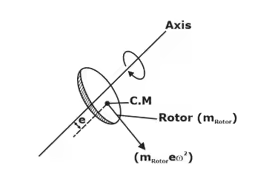At time, t
fun in a particular direction,
fun = (MRotor2)
where, f0 = (mRotor2) — max volume of unbalanced force.
ω — force frequency or excitation.

2. Reciprocating Unbalance: (in Piston-crank)
fun = mR2 sinθ
[mR—mass of Reciprocating Ports]
(mass of Piston + mass of crosshead + mass of connecting Rod)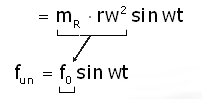Where, ω = forced frequency and m = machine mass (whole) which is under vibrations.

3. Forced-Damped Systems (Perfect Reality)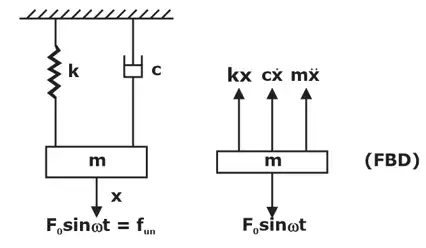Forced vibrations of a damped spring mass system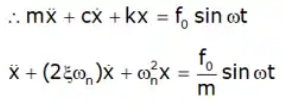This is the equation of forced-Damped System.
∴ The solution will be, x = c · f + P · I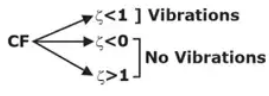After some time, CF = 0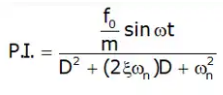After solving,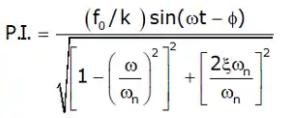Amplitude of forced vibrations (A):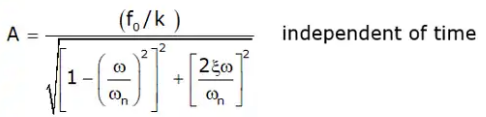Hence, x = PI
∴ x = A sin(ωt - ϕ)
Where,  A = Amplitude of steady state vibrations (independence of time) (forced vibrations)
Running system vibrations will never stop.
Every machine/mechanical running system must have one running life.

4. Magnification factor (M.F.)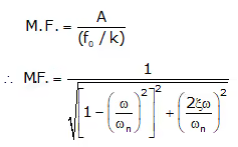∴ M.F. depends upon:
(i) ω/ωn
(ii) ζ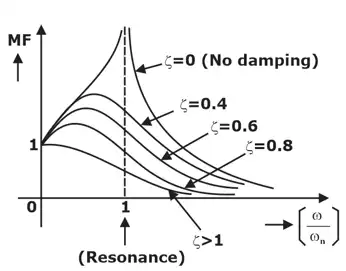Magnification Factor

• As Underdamping Increases
⇒ ζ↓
⇒ MF ↑ ⇒ A↑
⇒ Running life decrease ↓
• At Resonance
[ω/ωn = 1]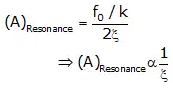Note: At some time 't',
F = f0sin[ωt]
x = Aω cos ωt
= Aω[sin{π/2 + (ωt - ϕ)}]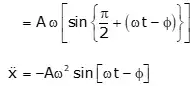The Basic Equation was,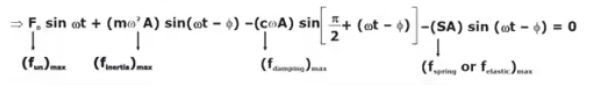5. Phase Diagram or forced-Damped System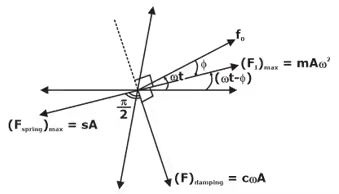• Spring and damping force’s max values are perpendicular to each other.
• Inertia force lies exactly opposite (At 180°) To the spring force’s max value.

6. Vibration Isolations
It is used to isolate the ground from the vibrations of the Running machine so as to save other stationary m/cs from these vibration effects.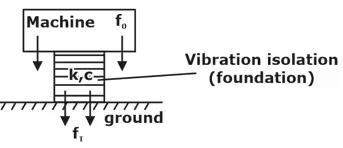Vibration Isolation

Fτ = force transmitted to the ground
Fτ < < < < < F0
∈ = Fτ / F0 = Transmissivity
0 < ∈ < 1
∈ → 0 (for Belts)
Now, Fτ = Resultant of forces of spring force and damping force (max values)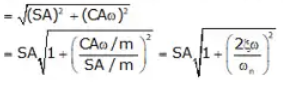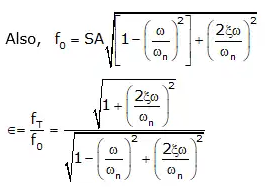Hence, ′∈′ depends upon:
(i) ω/ωn
(ii) ζ
Note: If, w/wn = 0 w/wn = √2 ⇒ ∈ = 1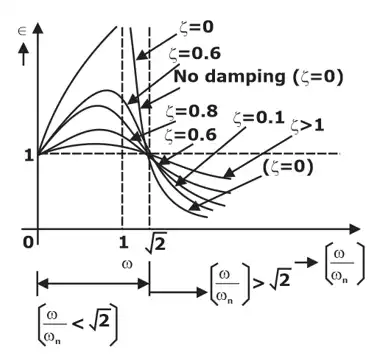• As Underdamping ↑ ⇒ ζ↓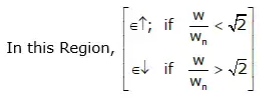∈ will be constant, if w/wn = √2
• Vibration Isolation is Effective
When ∈ < 1
⇒ (w/wn > √2) → called "Effective Vibration-isolation-zone".
• In Effective Vibration Isolation Zone:
w/wn > √2; Then At ζ = 0, e is minimum.
∴ No damping → is Best. (∵ = ∈ → 0 Best)
Hence, Damping is dangerous in this Zone.
⇒ Ass ↑ (in this zone). ∈↑ ⇒ fτ
Offer running on EduRev: Apply code STAYHOME200 to get INR 200 off on our premium plan EduRev Infinity!

## Theory of Machines (TOM)

94 videos|41 docs|28 tests

,

,

,

,

,

,

,

,

,

,

,

,

,

,

,

,

,

,

,

,

,

;